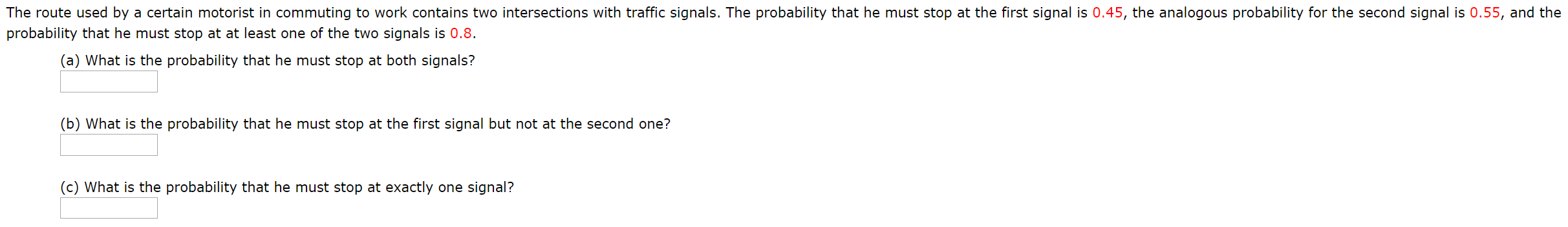# The route used by a certain motorist in commuting to work contains two intersections with traffic signals. The probability that he must stop at the first signal is 0.45, the analogous probability for the second signal is 0.55, and theprobability that he must stop at at least one of the two signals is 0.8.(a) What is the probability that he must stop at both signals?(b) What is the probability that he must stop at the first signal but not at the second one?(c) What is the probability that he must stop at exactly one signal?

Question
30 viewshelp_outlineImage TranscriptioncloseThe route used by a certain motorist in commuting to work contains two intersections with traffic signals. The probability that he must stop at the first signal is 0.45, the analogous probability for the second signal is 0.55, and the probability that he must stop at at least one of the two signals is 0.8. (a) What is the probability that he must stop at both signals? (b) What is the probability that he must stop at the first signal but not at the second one? (c) What is the probability that he must stop at exactly one signal? fullscreen
check_circle

Step 1

### Want to see the full answer?

See Solution

#### Want to see this answer and more?

Solutions are written by subject experts who are available 24/7. Questions are typically answered within 1 hour.*

See Solution
*Response times may vary by subject and question.
Tagged in
MathStatistics

### Other